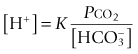# Hydrogen ion homoeostasis and blood gases

Chapter 3 Hydrogen ion homoeostasis and blood gases

# Clinical and Laboratory Assessment of Hydrogen Ion Status

It is vital that air is excluded from the syringe, both before and after drawing blood, and that, if possible, analysis is performed immediately. (In practice, the analysers are usually situated close to the patient, e.g. in intensive therapy units, rather than in a remote laboratory (see p. 5).) If the blood sample has to be transported, the syringe, capped with a blind hub and enclosed in a plastic bag, should be chilled in ice water. The analysers measure [H+] (strictly, activity), Pco2 and Po2 using specific electrodes; these measurements are together known colloquially as ‘blood gases’; they frequently measure other analytes in addition, for example, lactate, haemoglobin, sodium and potassium.

By the law of mass action, it follows from the equations describing the dissociation of carbonic acid (Equations 3.1 and 3.2) that [H+] is directly proportional to Pco2 and inversely proportional to bicarbonate concentration; that is, it is determined by the ratio of Pco2 to bicarbonate:(3.5)

The constant, K, embraces the dissociation constants for Equations 3.1 and 3.2 and the solubility coefficient of carbon dioxide, which governs the concentration of the gas in solution at a given partial pressure. When [H+] is measured in nmol/L, bicarbonate in mmol/L and Pco2 in kilopascals (kPa), the value of K is approximately 180 at 37°C; if Pco2 is measured in mm Hg, the value of K is 24.

It follows that it is possible to calculate the bicarbonate concentration from the [H+] and Pco2 alone. In blood gas analysers, the bicarbonate concentration is derived by calculation and not measured. It is not the same as the bicarbonate (strictly, total carbon dioxide) measured by most laboratory analysers. There has been considerable argument over whether it is valid to derive a bicarbonate concentration in this way, given that the values of the constants involved are based on observations in supposedly ideal solutions, which biological fluids are not. However, for most practical purposes the derivation is an acceptable one.

An appreciation of the relationship between [H+], bicarbonate concentration and Pco2 is of fundamental importance to an understanding of the pathophysiology of hydrogen ion homoeostasis. It will be apparent from Equation 3.5 that the relationships between [H+] and Pco2 and between bicarbonate concentration and Pco2 are linear. These relationships have been quantified by measurements made in vivo, and it is therefore possible to predict the effect of a change in one variable on another, for example the effect of an acute rise in Pco2 on [H+]. This information is an important aid in the interpretation of acid–base data.

The relationships between [H+], Pco2 and bicarbonate concentration are plotted in Figure 3.5. This diagram may be useful as an aide-mémoire to the interpretation of acid–base data but should not be used as a substitute for a full understanding of the underlying principles.

# Disorders of Hydrogen Ion Homoeostasis

## Non-respiratory (metabolic) acidosis

The primary abnormality in non-respiratory acidosis is either increased production or decreased excretion of hydrogen ions other than from carbon dioxide. In some cases, both may contribute. Loss of bicarbonate from the body can also, indirectly, cause an acidosis. Causes of non-respiratory acidosis are given in Figure 3.6. Excess hydrogen ions are buffered by bicarbonate (Equation 3.2) and other buffers. The carbonic acid thus formed dissociates (Equation 3.1) and the carbon dioxide is lost in the expired air. This buffering limits the potential rise in hydrogen ion concentration, but at the expense of a reduction in bicarbonate concentration, which is a constant feature of non-respiratory acidosis.

Compensation is effected by hyperventilation, which increases the removal of carbon dioxide and lowers the Pco2. The Pco2/[HCO3] ratio falls, thus tending to reduce the [H+] (Equation 3.5). Hyperventilation is a direct result of the increased [H+] stimulating the respiratory centre. Respiratory compensation cannot completely normalize the [H+], as it is the high concentration itself that stimulates the compensatory hyperventilation. Furthermore, the increased work of the respiratory muscles produces carbon dioxide, thereby limiting the extent to which the Pco2 can be lowered.

If the cause of the acidosis is not corrected, a new steady state may be attained, with a raised [H+], low bicarbonate and low Pco2. In the steady state, the decrease in Pco2 attributable to respiratory compensation is approximately 0.17 kPa (1.3 mm Hg) for each 1 mmol/L decrement in bicarbonate concentration. The extent to which compensation can take place will be limited if respiratory function is compromised. Even with normal respiratory function, it is exceptional for a Pco2 of <1.5 kPa (11.3 mm Hg) to be recorded, however severe the non-respiratory acidosis.

The complete correction of a non-respiratory acidosis requires reversal of the underlying cause, for example rehydration and insulin for diabetic ketoacidosis (see Case history 11.2) and removal of salicylate in salicylate overdose. It is important to maintain adequate renal perfusion to maximize renal hydrogen ion excretion. The use of exogenous bicarbonate to buffer hydrogen ions is discussed below and on p. 193.

### Decreased excretion of hydrogen ions

Acidosis occurs in renal glomerular failure (see Case history 4.2), when the decreased glomerular filtration causes a reduction in the amount of sodium that is filtered and, therefore, available for exchange with hydrogen ions. The amount of phosphate filtered and available for buffering also decreases. Renal tubular acidoses are discussed in Chapter 4.

Apr 3, 2019 | Posted by in BIOCHEMISTRY | Comments Off on Hydrogen ion homoeostasis and blood gases
Premium Wordpress Themes by UFO Themes